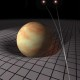Home » Cosmology » Extending the BGV Theorem to Cosmogonic Models Positing an Infinite Contraction

# Extending the BGV Theorem to Cosmogonic Models Positing an Infinite Contraction

### Recent CommentsNathan on Calculating the Probability of…Nathan on Calculating the Probability of…Jim on Calculating the Probability of…Jack Spell on The Solution to FatalismFrederick on The Solution to Fatalism
Advertisements

You all will have to forgive me for making such a technical post as this blog’s first; it just so happens that this has been the topic of my recent research. Sorry.

For those of you who do not know, there was a kinematic incompleteness theorem (http://arxiv.org/abs/gr-qc/0110012v2) proven in 2003 that (in very non-technical terms) demonstrates that any universe that has been, on average, expanding throughout its history, cannot be eternal to the past, but must have an past spacetime boundary (i.e., a beginning). Well, it just so happens that our universe has been, by all appearances, expanding throughout its history.

However, there have been many attempts to craft highly speculative cosmogonic models that evade the Borde-Guth-Vilenkin theorem (hereafter, BGV). One of which posits an infinite contraction prior to a bounce, followed by a subsequent contraction phase. It is my contention that such a model does not in fact evade the theorem, and I shall engage in an endeavor to articulate why I believe BGV to conflict withspacetimes in this post. I want to first lay out some basics:

1.      A spacetime is past-incomplete if there is a null (or timelike) geodesic maximally extended to the past that is finite in length.

2.      As long as the expansion rate averaged over the affine parameter λ along a geodesic is positive (), BGV proves that there will be causal geodesics that, when extended to the past of an arbitrary point, reach the boundary of the inflating region of spacetime in a finite proper time τ (finite affine length, in the null case).

3.      The measure of temporal duration fromis a quantity that is actually infinite () rather than potentially infinite ().

Now, if the velocity of a geodesic observer(relative to commoving observers in an expanding congruence) in an inertial reference frame is measured at an arbitrary timeto be any finite nonzero value, then she will necessarily reach the speed of light at some timeand the intervalwill be have a finite value. If an infinite contraction preceded the “bounce” (and indeed it must do so necessarily ifis to be avoided), then the time coordinatewill run monotonically fromas spacetime contracts during, bounces at, then expands for allThus, if what I have argued above is correct, then the implications are unmistakable: as long as:

1.  is a non-comoving geodesic observer;
2.  is in an inertial reference frame;
3.  is moving from;
4.  has been tracing a contracting spacetime where;

it therefore follows that the relative velocity ofwill get faster and faster as she approaches the bounce at. Moreover, since we know thatwill reach the speed of light in a finite proper time, coupled with the fact that the intervalis infinite, we can be sure the she will reach the speed of light well-before ever making it to the bounceand therefore cannot be geodesically complete.

Advertisements